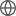Old Web
English
The 2mm Mapping Obscuration to Reionization with ALMA (MORA) Survey was designed to detect high redshift ($z\gtrsim4$), massive, dusty star-forming galaxies (DSFGs). Here we present two, likely high redshift sources, identified in the survey whose physical characteristics are consistent with a class of optical/near-infrared (OIR) invisible DSFGs found elsewhere in the literature. We first perform a rigorous analysis of all available photometric data to fit spectral energy distributions and estimate redshifts before deriving physical properties based on our findings. Our results suggest the two galaxies, called MORA-5 and MORA-9, represent two extremes of the "OIR-dark" class of DSFGs. MORA-5 ($z_{\rm phot}=4.3^{+1.5}_{-1.3}$) is a significantly more active starburst with a star-formation rate of 830$^{+340}_{-190}$M$_\odot$yr$^{-1}$ compared to MORA-9 ($z_{\rm phot}=4.3^{+1.3}_{-1.0}$) whose star-formation rate is a modest 200$^{+250}_{-60}$M$_\odot$yr$^{-1}$. Based on the stellar masses (M$_{\star}\approx10^{10-11}$M$_\odot$), space density ($n\sim(5\pm2)\times10^{-6}$Mpc$^{-3}$, which incorporates two other spectroscopically confirmed OIR-dark DSFGs in the MORA sample at $z=4.6$ and $z=5.9$), and gas depletion timescales ($<1$Gyr) of these sources, we find evidence supporting the theory that OIR-dark DSFGs are the progenitors of recently discovered \$3